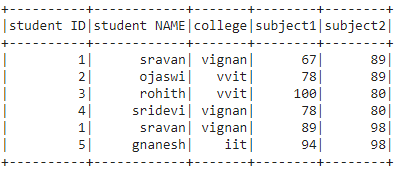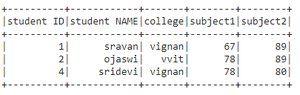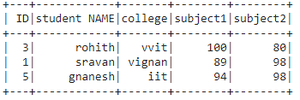# How to select a range of rows from a dataframe in PySpark ?

• Last Updated : 18 Jul, 2022

In this article, we are going to select a range of rows from a PySpark dataframe.

It can be done in these ways:

• Using filter().
• Using where().
• Using SQL expression.

Creating Dataframe for demonstration:

## Python3

 `# importing module``import` `pyspark` `# importing sparksession from pyspark.sql module``from` `pyspark.sql ``import` `SparkSession` `# creating sparksession and giving an app name``spark ``=` `SparkSession.builder.appName(``'sparkdf'``).getOrCreate()` `# list  of students  data``data ``=` `[[``"1"``, ``"sravan"``, ``"vignan"``, ``67``, ``89``],``        ``[``"2"``, ``"ojaswi"``, ``"vvit"``, ``78``, ``89``],``        ``[``"3"``, ``"rohith"``, ``"vvit"``, ``100``, ``80``],``        ``[``"4"``, ``"sridevi"``, ``"vignan"``, ``78``, ``80``],``        ``[``"1"``, ``"sravan"``, ``"vignan"``, ``89``, ``98``],``        ``[``"5"``, ``"gnanesh"``, ``"iit"``, ``94``, ``98``]]` `# specify column names``columns ``=` `[``'student ID'``, ``'student NAME'``,``           ``'college'``, ``'subject1'``, ``'subject2'``]` `# creating a dataframe from the lists of data``dataframe ``=` `spark.createDataFrame(data, columns)` `# display dataframe``dataframe.show()`

Output:Method 1: Using filter()

This function is used to filter the dataframe by selecting the records based on the given condition.

Syntax: dataframe.filter(condition)

Example: Python code to select the dataframe based on subject2 column.

## Python3

 `# select dataframe between``# 23 and 78 marks in subject2``dataframe.``filter``(``  ``dataframe.subject1.between(``23``,``78``)).show()`

Output:Method 2: Using where()

This function is used to filter the dataframe by selecting the records based on the given condition.

Syntax: dataframe.where(condition)

Example 1: Python program to select dataframe based on subject1 column.

## Python3

 `# select dataframe between``# 85 and 100 in subject1 column``dataframe.where(``  ``dataframe.subject1.between(``85``,``100``)).show()`

Output:Example 2: Select rows in dataframe by college column

## Python3

 `# select dataframe in college column``# for vvit``dataframe.where(``  ``dataframe.college.between(``"vvit"``,``"vvit"``)).collect()`

Output:

[Row(ID=’2′, student NAME=’ojaswi’, college=’vvit’, subject1=78, subject2=89),

Row(ID=’3′, student NAME=’rohith’, college=’vvit’, subject1=100, subject2=80)]

Method 3: Using SQL Expression

By using SQL query with between() operator we can get the range of rows.

Syntax: spark.sql(“SELECT * FROM my_view WHERE column_name between value1 and value2”)

Example 1: Python program to select rows from dataframe based on subject2 column

## Python3

 `# create view for the dataframe``dataframe.createOrReplaceTempView(``"my_view"``)` `# data subject1 between 23 and 78``spark.sql("SELECT ``*` `FROM my_view WHERE\``subject1 between ``23` `and` `78``").collect()`

Output:

[Row(student ID=’1′, student NAME=’sravan’, college=’vignan’, subject1=67, subject2=89),

Row(student ID=’2′, student NAME=’ojaswi’, college=’vvit’, subject1=78, subject2=89),

Row(student ID=’4′, student NAME=’sridevi’, college=’vignan’, subject1=78, subject2=80)]

Example 2: Select based on ID

## Python3

 `# create view for the dataframe``dataframe.createOrReplaceTempView(``"my_view"``)` `# data subject1 between 23 and 78``spark.sql("SELECT ``*` `FROM my_view WHERE\``ID` `between ``1` `and` `3``").collect()`

Output:

[Row(ID=’1′, student NAME=’sravan’, college=’vignan’, subject1=67, subject2=89),

Row(ID=’2′, student NAME=’ojaswi’, college=’vvit’, subject1=78, subject2=89),

Row(ID=’3′, student NAME=’rohith’, college=’vvit’, subject1=100, subject2=80),

Row(ID=’1′, student NAME=’sravan’, college=’vignan’, subject1=89, subject2=98)]

My Personal Notes arrow_drop_up